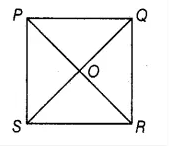# PQRS is a square.`
Question:

PQRS is a square. PR and SQ intersect at O. Then ∠POQ is a

(a) Right angle

(b) Straight angle

(c) Reflex angle

(d) Complete angle

Solution:

(a) Right angleThe diagonals in the square intersect each other at right angle i.e. 90o

Therefore, ∠POQ is a right angle.